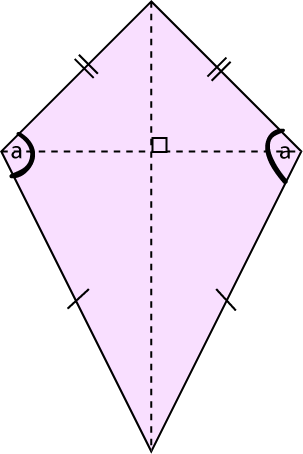# Definition of KiteA kite is a quadrilateral (4-sided flat shape) which has the following properties:

• It has two pairs of adjacent sides that are equal in length (we can also find two pairs of adjacent sides that have unequal lengths).
• Its diagonals are at right angles to each other.
• One diagonal cuts the other diagonal in half.
• The angles (labelled $a$ in the picture) between the unequal adjacent sides are equal.

### Description

The aim of this dictionary is to provide definitions to common mathematical terms. Students learn a new math skill every week at school, sometimes just before they start a new skill, if they want to look at what a specific term means, this is where this dictionary will become handy and a go-to guide for a student.

### Audience

Year 1 to Year 12 students

### Learning Objectives

Learn common math terms starting with letter K

Author: Subject Coach
You must be logged in as Student to ask a Question.